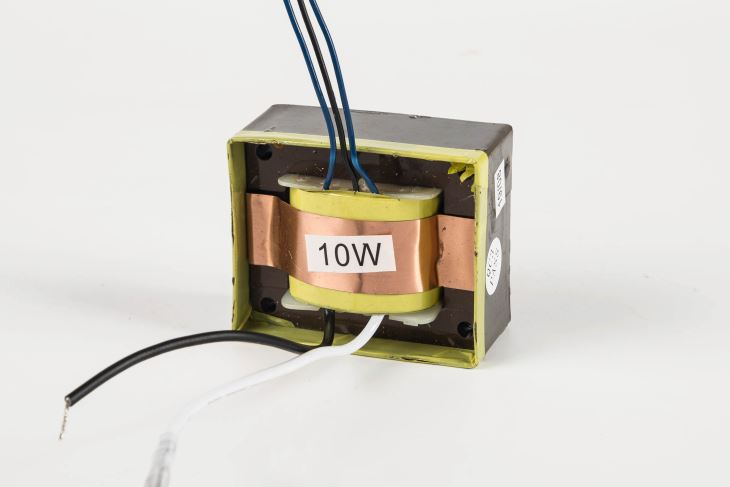0755-29413561
139 2374 6208

工作时间

联系方式分享到：

# EI6613.5 电源变压器

 产品详情低频变压器用来传播信号电压和信号功率，还可实现电路之间的阻抗匹配，对直流电具有隔离作用。高频变压器与低频变压器原理上没区别。但由于高频和低频的频率不同，变压器所用的铁芯不同。低频变压器一般用高导磁率的硅钢片，高频变压器则用高频铁氧体磁芯。低频变压器分类它分为级间耦合变压器、输入变压器和输出变压器，外形均于电源变压器相似。1．极间耦合变压器：级间耦合变压器用在两级音频放大电路之间，作为耦合元件，将前级放大电路的输出信号传送至后一级，并作适当的阻抗变换。2．输入变压器：在早期的半导体收音机中，音频推动级和功率放大级之间使用的变压器为输入变压器，起信号耦合、传输作用，也称为推动变压器。输入变压器有单端输入式和推挽输入式。若推动电路为单端电路，则输入变压器也为单端输入式变压器；若推动电路为推挽电路，则输入变压器也为推挽输入式变压器。3．输出变压器：输出变压器接功率放大器的输出电路与扬声器之间，主要起信号传输和阻抗匹配的作用。输出变压器也分为单端输出变压器和推挽输出变压器两种。低频变压器原理舌口32 mm、厚34 mm、宽96 mm，最大功率使用要多粗的线，舌口是指，EI型变压器铁芯截面积是指E片中间那一横(插入Satons变压器骨架中间方口里的)的宽度即铁芯舌宽与插入变压器骨架方口里所有E片的总厚度即叠厚的乘积最简单的就是指变压器骨架中间方口的面积，变压器铁芯截面积是指线圈所套着的部分：舌宽×叠厚=截面积，单位：cm2。 第一种计算方法(1)变压器矽钢片截面：3.2 cm*3.4cm*0.9=9.792cm2(2)根据矽钢片截面计算变压器功率：P=S/K^2=(9.79/1.25)^2=61.34瓦(取60瓦)(3)根据截面计算线圈每伏几匝：W=4.5*105/BmS=4.5*105/(10000*9.79)=4.6匝/伏(4)初级线圈匝数：220*4.6=1012匝(5)初级线圈电流：60W/220V=0.273A(6)初级线圈线径：d=0.715 =0.37(mm)(7)次级线圈匝数：2*(51*4.6*1.03)=2*242(匝)(1.03是降压系素，双级51V=2*242匝)(8)次级线圈电流：60W/(2*51V)=0.59A(9)次级线径：d=0.715 =0.55(mm)第二种计算方法E形铁芯以中间舌为计算舌宽的。计算公式：输出功率：P2=UI考虑到变压器的损耗，初级功率：P1=P2/η(其中η=0.7～0.9，一般功率大的取大值)每伏匝数计算公式：N(每伏匝数)=4.5×105/B×S(B=硅钢片导磁率，一般在8000～12000高斯，好的硅钢片选大值，反之取小值。S=铁芯舌的面积，单位是cm2)如硅钢片质量一般可选取10000高斯，那么可简化为：N=45/S计算次级绕组圈数时，考虑变压器漏感和导线铜损，须增加5% 绕组余量。初级不用加余量。由电流求线径：I=P/U (I=A，P=W，U=V)以线径每平方毫米≈2.5～2.6A选取。第三种计算方法首先要说明的是变压器的截面积是线圈所套住位置的截面积。如果你的铁心面积(线圈所套住位置)为32*34=1088 mm2=10.88 cm2小型变压器的简易计算：1，求每伏匝数每伏匝数=55/铁心截面例如，你的铁心截面=3.5╳1.6=5.6平方厘米故，每伏匝数=55/5.6=9.8匝2，求线圈匝数初级线圈 n1=220╳9.8=2156匝次级线圈 n2=8╳9.8╳1.05=82.32，可取为82匝次级线圈匝数计算中的1.05是考虑有负荷时的压降3，求导线直径你未说明你要求输出多少伏的电流是多少安?这里我假定为8V，电流为2安。变压器的输出容量=8╳2=16伏安变压器的输入容量=变压器的输出容量/0.8=20伏安初级线圈电流I1=20/220=0.09安导线直径 d=0.8√I初级线圈导线直径 d1=0.8√I1=0.8√0.09=0.24毫米次级线圈导线直径 d2=0.8√I2=0.8√2=1.13毫米要注意层间电压绝缘，引出端绝缘问题。

联系方式